Links to Other Magic Square Pages:
Odd Magic Squares
Even Magic Squares

# Singly Even Magic Squares (Part 3)

Singly even magic squares are one of the three types of magic square.

The other two types are:
odd (n=3, 5, 7, 9, 11, etc.) and
doubly even (multiple of 4 where n=4, 8, 12, 16, 20, etc.)

#### Singly Even Magic Squares

Well it seems we have encountered another phrase that isn't very descriptive. Basically, "singly even" means divisible by 2 but not by four. A formula for generating singly even numbers is (n*4) + 2, which generates the numbers 2, 6, 10, 14, 18, 22, 26, 30 and so on. There is no magic square that can be constructed in a 2 by 2 square but singly even magic squares can be constructed for n=6, 10, 14 and so on.

Singly even magic squares are the most difficult to construct and so let's start with the smallest possible one where n = 6.
To get the sum, we'll use the formula that we previously used: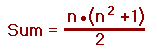So, the sum for n=6 is 111.

The first step is to break the square into four smaller, equally-sized squares. So, for constructing a 6 by 6 magic square we start with four 3 by 3 squares. We then construct four magic squares in a pattern indicated here: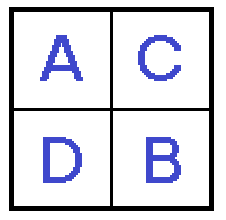Basically, this means that in section 'A' we will build a magic square with the numbers 1 through 9, in section 'B' the magic square will start with 10 and end with 18, section 'C' will have the numbers 19 through 27 and section 'D' goes from 28 through 36. So, when we finish this step, the square looks like this: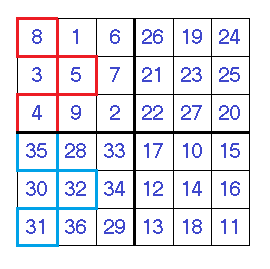Well, you probably noticed that six numbers on the left side of the square have been highlighted in red or blue and that's because there is still some more work to be done on this magic square.
In this case, the "red" numbers have to be moved where the "blue" numbers are and vice versa. After doing this, the square should now look like this: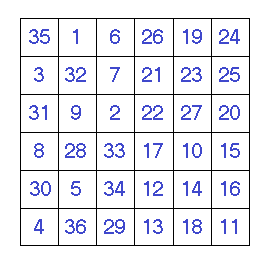Yes, finally we are done and all rows, columns and both diagonals sum to 111.

Next, we'll move on to building a 10 by 10 square. The rules about building four n ÷ 2 squares in the "ABCD" pattern still apply but if you notice, in the left side square, the numbers that need shifting make up a different pattern from the 6 by 6 square.
The numbers on the left side that require shifting are one column wider and for the first time we have a right hand column to deal with.The square on the right side is the completed square after all those numbers have been shifted and all rows, columns and diagonals sum to 505.

We can see that the pattern for the cells that need to be shifted is now forming a very predictable pattern.
Perhaps by building a 14 by 14 magic square we could see if the pattern continues in the same fashion.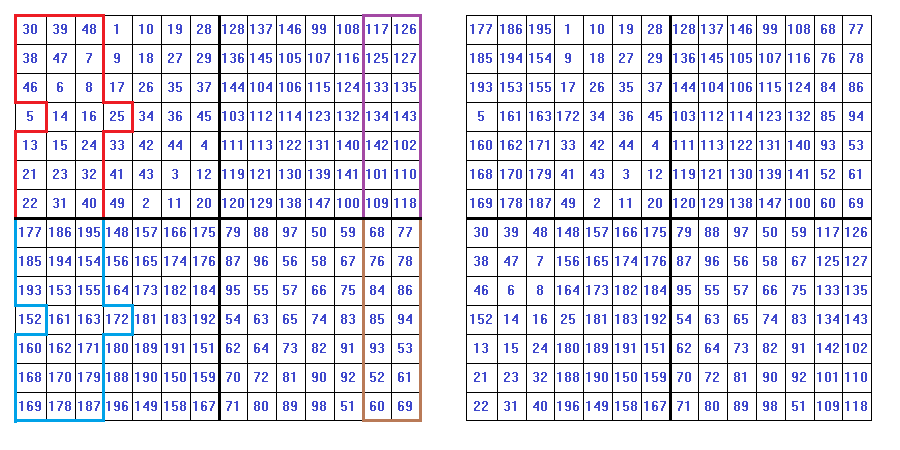Comparing this to the 10 by 10 square, we can see the pattern is now quite simple. The cells that require shifting for the 14 by 14 square are one column greater than the 10 by 10 square. The magic sum for this square is 1,379.

To make absolutely sure that the pattern for the shifted cells remains the same, let's construct a singly even magic square for n=30 which will have a magic sum of 13,515.
The square after the first step is illustrated here: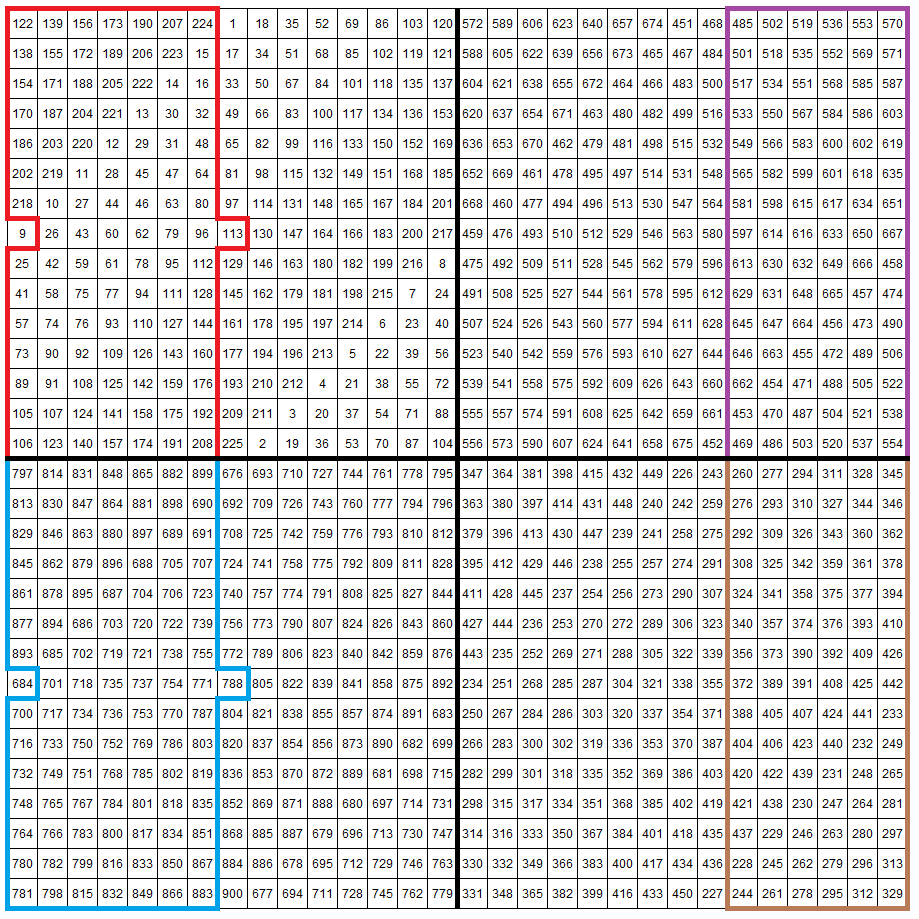After shifting the cells, the completed magic square now looks like this: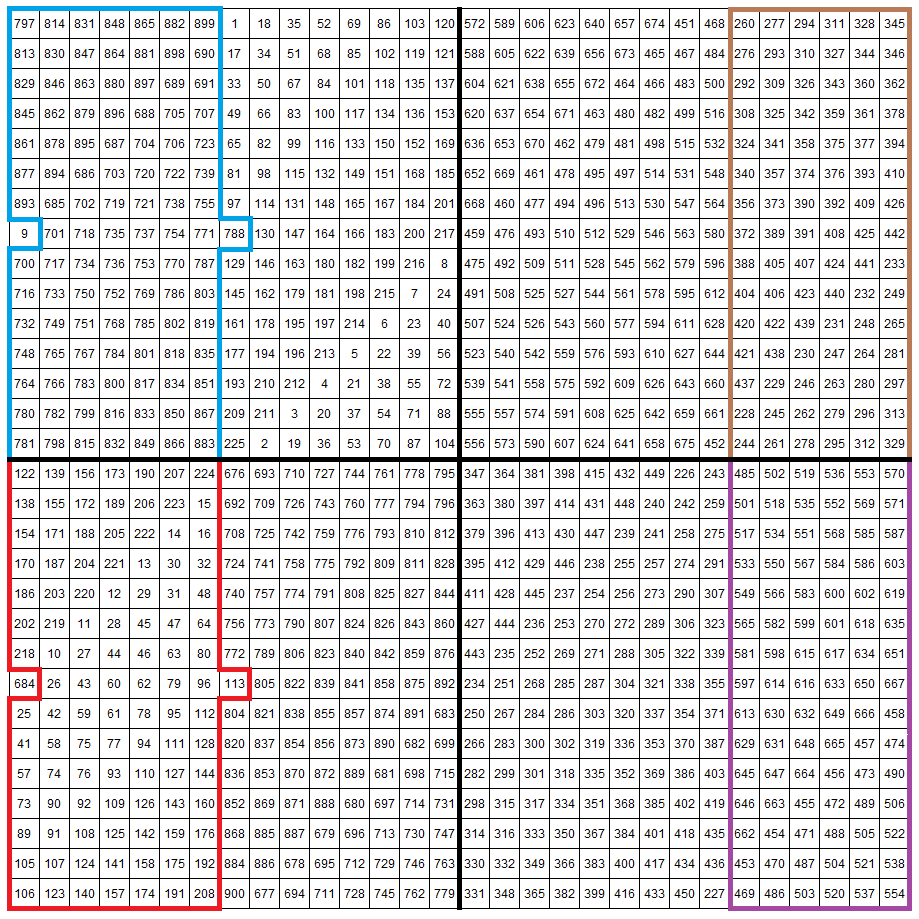To help you identify the shifted cells, we have highlighted these in color in the "before" and "after" graphics.
Now that you are very sure of how to construct singly even magic squares, you can go on to create ones for n=26, n=34 or a great many other sizes.